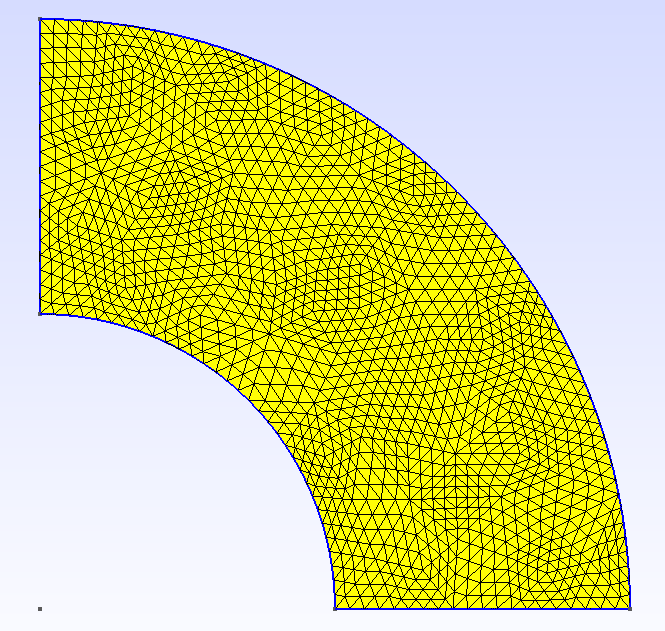# Benchmark for Mixed Poisson equations

The goal of this section is to test the convergence of the HDG algorithm for the mixed poisson problem in 2D and 3D domain.

\begin{equation} \left\{ \begin{aligned} \mathbf{u} + k\nabla p &= 0 &\text{ in } \Omega\\ \nabla\cdot\mathbf{u} &= f &\text{ in } \Omega\\ p &= g_D &\text{ on } \Gamma_D\\ \mathbf{u}\cdot\mathbf{n} &= g_N &\text{ on } \Gamma_N\\ \int_{\Gamma_I} \mathbf{u}\cdot\mathbf{n} &= g_I &\text{ on } \Gamma_I \end{aligned} \right. \end{equation}

The exact solutions and domain are presented in the following figures for 2D and 3D.
We have tested three cases:

• only Dirichlet conditions:

\begin{equation*} \Gamma_D=\partial\Omega\text{ and }\Gamma_N=\Gamma_I=\emptyset \end{equation*}
• only Neumann conditions:

\begin{equation*} \Gamma_N=\partial\Omega\text{ and }\Gamma_D=\Gamma_I=\emptyset \end{equation*}
• with integral boundary condition:

\begin{equation*} \Gamma_D=\{(x,y,z), y=0\}\text{, }\Gamma_I=\{(x,y,z), x=0\}\text{ and }\Gamma_N=\partial\Omega\setminus(\Gamma_D\cup\Gamma_I) \end{equation*}

For those tests, we uses the following parameters:

• $k=-1$

• $p=\frac{1}{2\pi}atan(y,x)$

• $u=\frac{1}{2\pi(x^2+y^2)}(y,-x)$

• $f=0$

On the following domain: